## How to calculate a correlation coefficient in Tableau

by Emily Dowling

What is a correlation coefficient?

A correlation coefficient is a value that quantifies the relationship of two or more variables. In linear correlation the coefficient quantifies the strength and direction of the correlation between the variables.

One type of correlation coefficient is the Pearson product-moment correlation coefficient, also known as r, which measures linear correlation and provides a value between -1 and +1.

• 1 is total positive correlation
• 0 is no correlation
• −1 is total negative correlation

Positive Correlation                                  No Correlation                                       Negative Correlation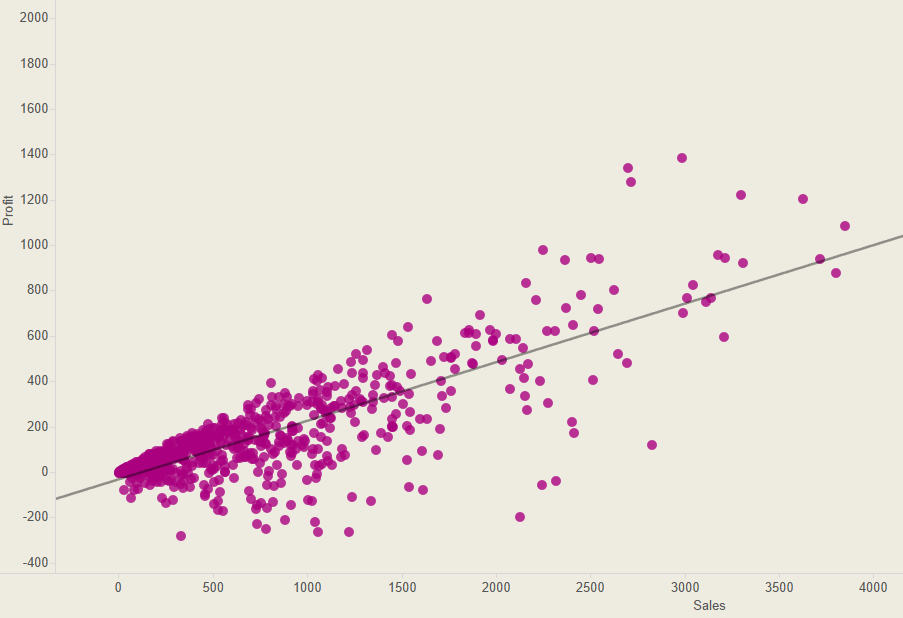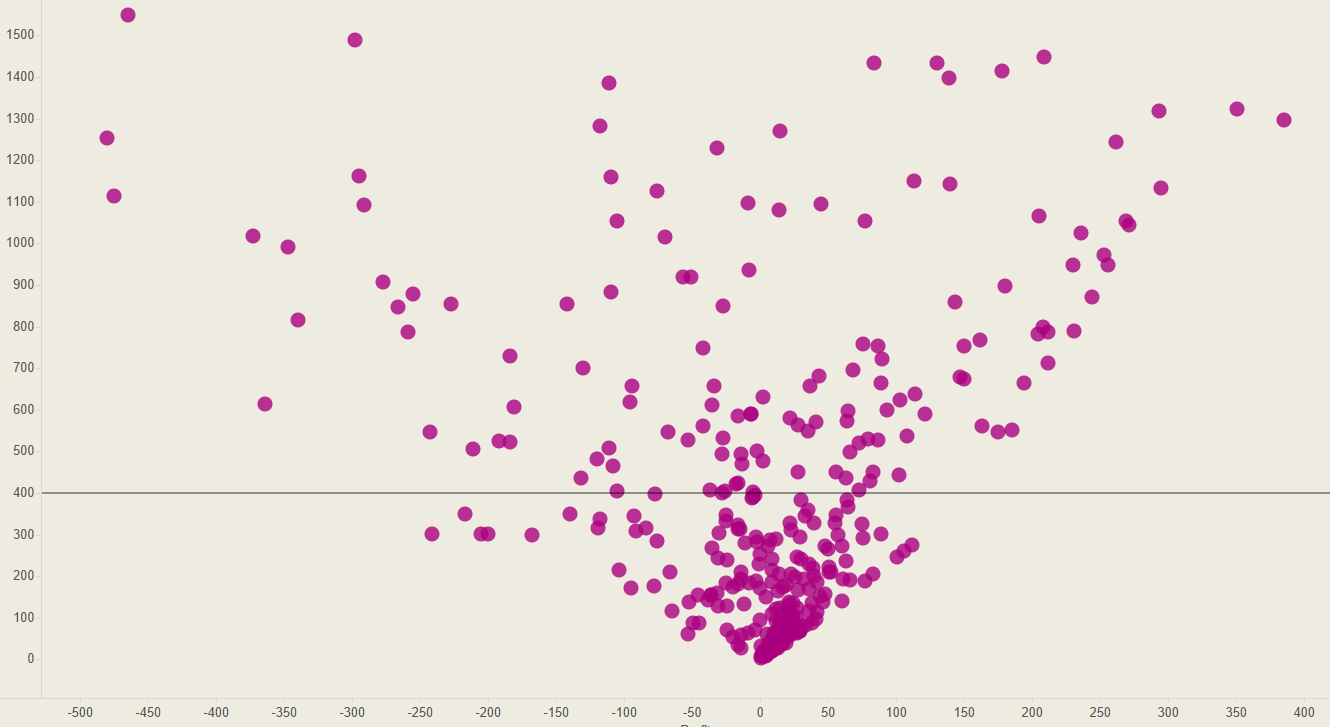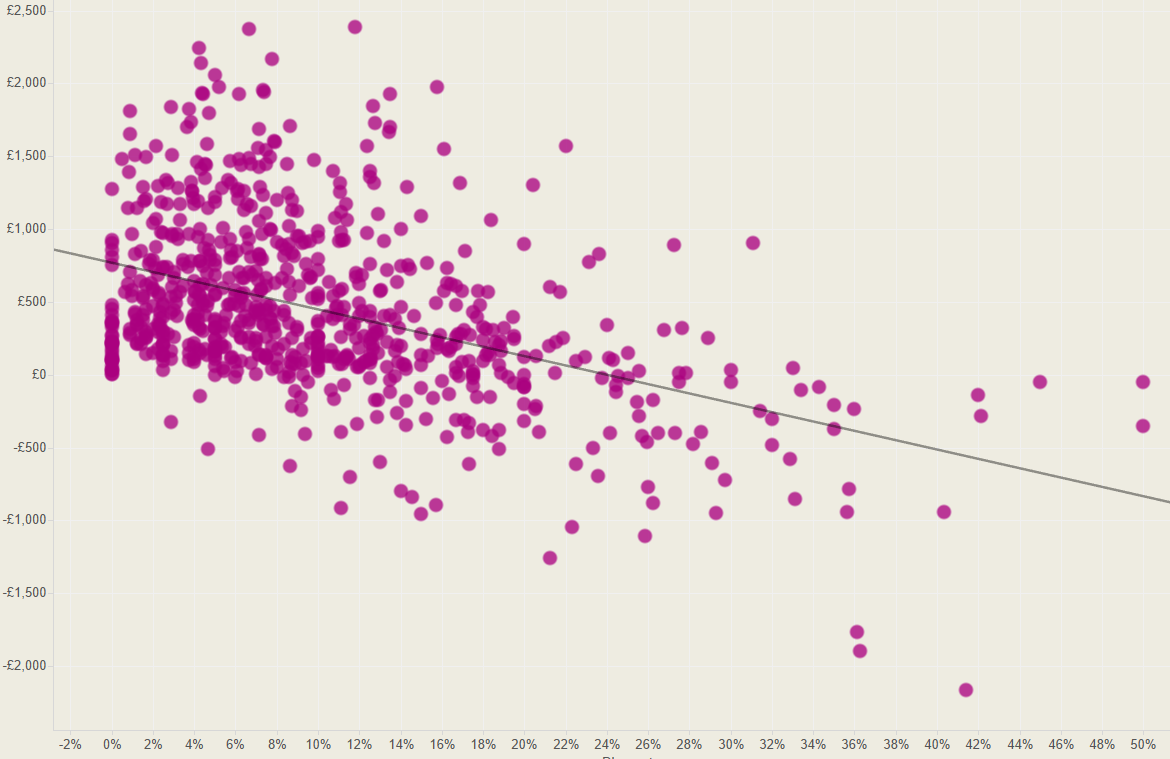If two variables have a strong positive correlation, then the r value will be nearer to +1; i.e.  x increases as y increases; if they have a strong negative correlation then the r value will be nearer to -1; i.e.  x increases as y decreases. A strong positive correlation is generally considered to be a value between +0.8 and +1; with anything below +0.5 considered to be weak.

How to calculate correlation coefficients

To measure R, the strength of a correlation, the covariance (the dependence between variables) needs to be determined and then divided by the product of the variables’ standard deviations. There are a few different types of formula to determine the correlation coefficient, I used the below formula, which for my data meant:

n = size

x = profit

y = sales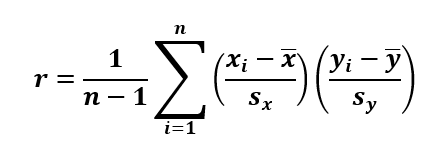This formula can appear pretty daunting, but once you break it down into parts and understand what each part means, it becomes much easier to translate this into a Tableau calculation.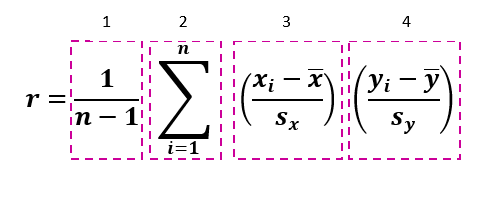Part 1: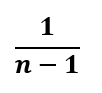Which simply means 1/(SIZE()-1)

Tableau already has a SIZE function which you can use.

Part 2: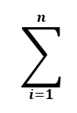We can just use the WINDOWSUM function in Tableau

Part 3: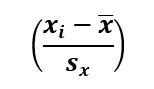For this part, xi = sum of profit, and  mean of profit, i.e. the window average of the sum of profit, and sx is the standard deviation of profit. Therefore we need to subtract the mean from the sum of profit and then divide that by the standard deviation:

(SUM([Profit])-WINDOW_AVG(SUM([Profit]))) / WINDOW_STDEV(SUM([Profit])))

Part 4: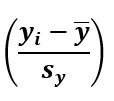Part 4 is exactly the same as part three except we need to swap profit for sales. i.e.

(SUM([Sales])-WINDOW_AVG(SUM([Sales]))) / WINDOW_STDEV(SUM([Sales])))

When you have all these parts, we need to multiply them by each other. It is crucial at this point to ensure that all the parentheses are in the correct places so that you get the correct r value.

So the final formula is:

1/(SIZE()-1) * WINDOW_SUM(( (SUM([Profit])-WINDOW_AVG(SUM([Profit]))) / WINDOW_STDEV(SUM([Profit]))) * (SUM([Sales])-WINDOW_AVG(SUM([Sales]))) / WINDOW_STDEV(SUM([Sales])))

So now to use this formula in Tableau.

I built a simple view (using Superstore Sales) to see if there was any correlation between sales and profits per category. I put Category and Sum(Profit) on Rows, Sum(Sales) on to Columns, and Customer Name on to Detail.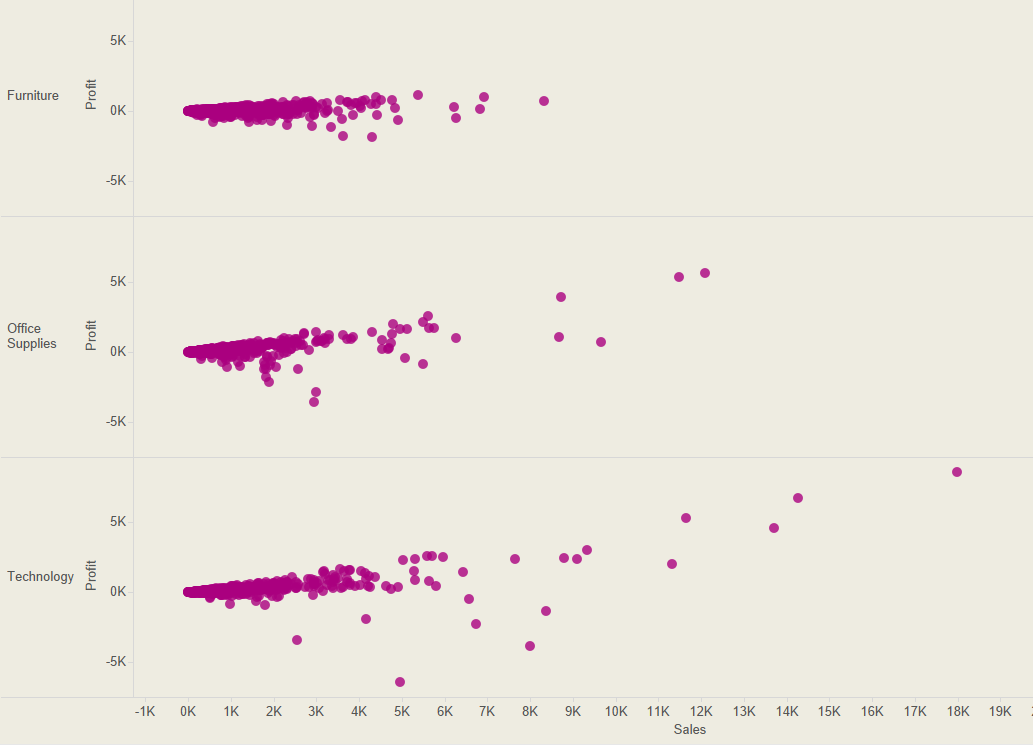Step 2: Create a calculated field

Next, I brought in my correlation coefficient formula and created a calculated field.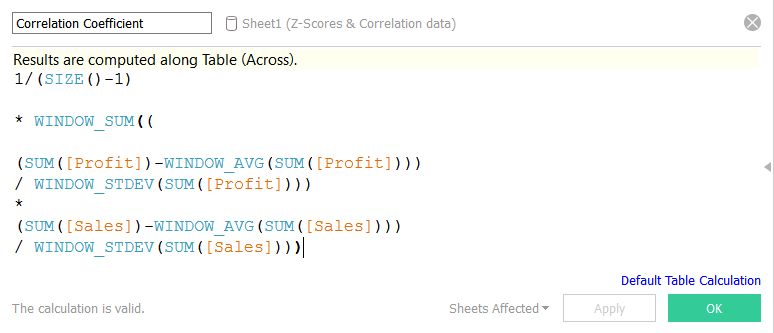Step 3: Drag to colour

Next I simply dragged my correlation coefficient calculation on to colour. However, similar to my Z-Scores formula, because this calculation using a Table Calculation, it needs to be computed by something. Therefore, we need to right click, select Compute Using, and click on (in this case) Customer Name (because that is what our view is detailing).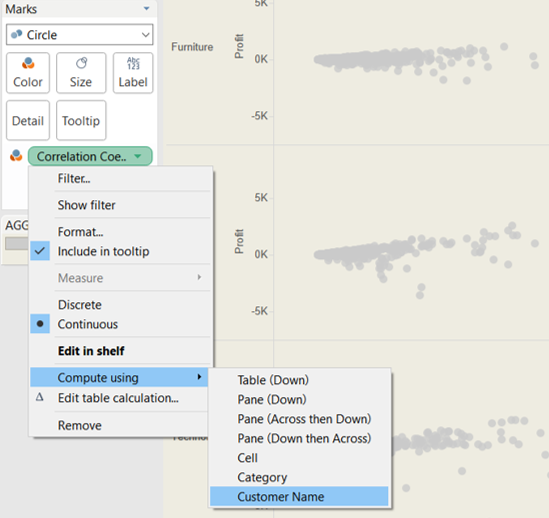Now we can see the strength of the correlation between profits and sales for each of the categories. The darker the colour the stronger the correlation.

A few final things that can be done: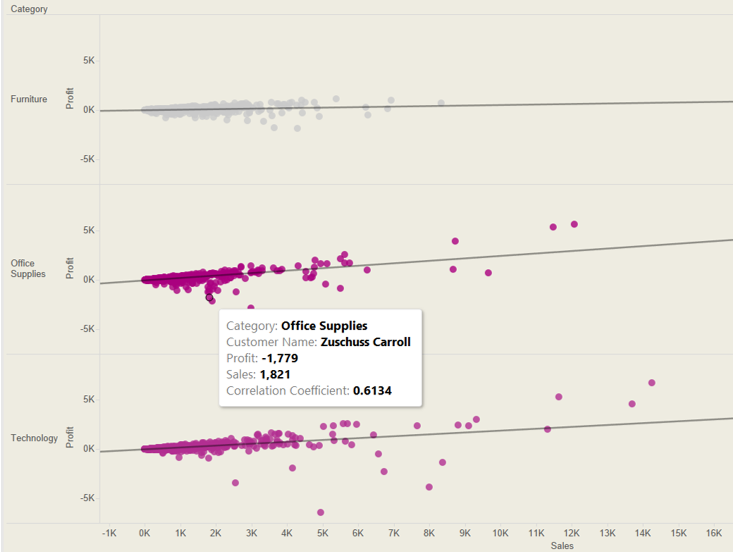• Bring other dimensions into the view, e.g. Region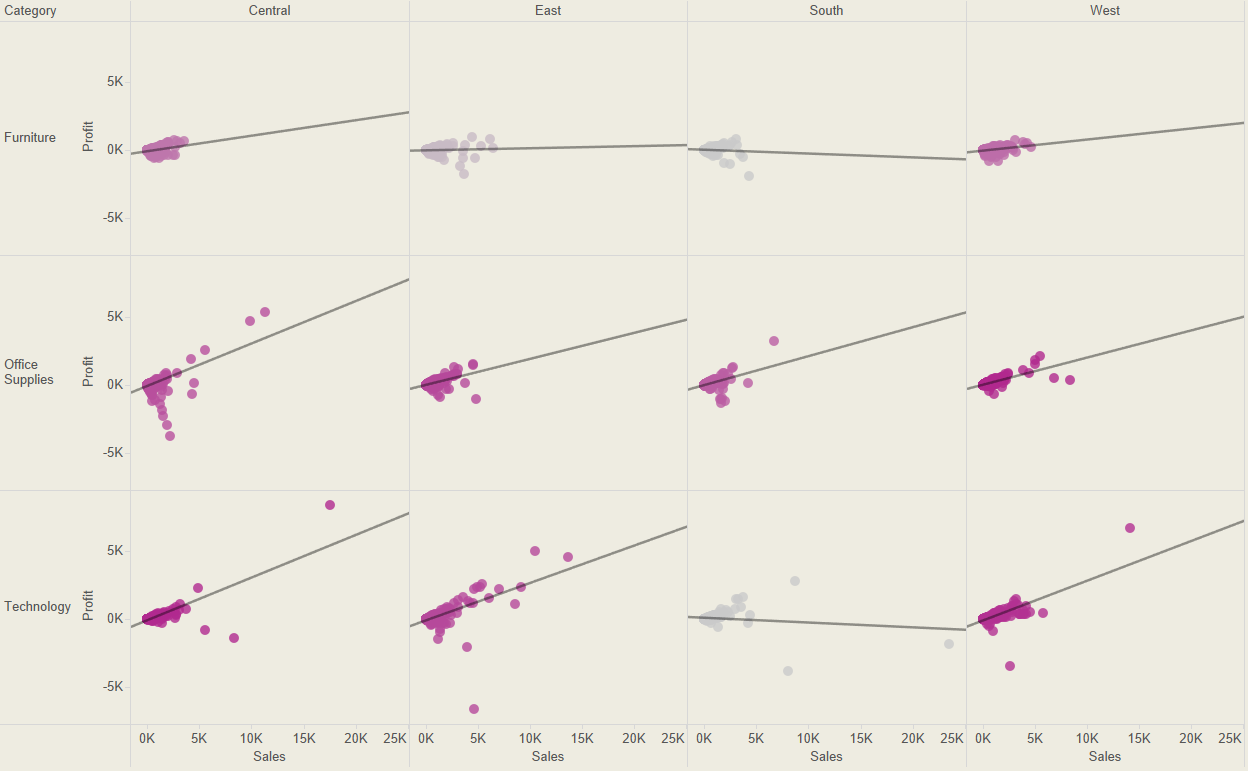Emily Dowling

Fri 02 Sep 2016

Mon 08 Aug 2016

Tue 02 Aug 2016

Thu 07 Jul 2016